# ATtiny1614 Function Generator

A tiny function generator built using the ATtiny1614 microprocessor and its internal Digital-To-Analog Convertor (DAC).

IntermediateFull instructions provided20 hours1,246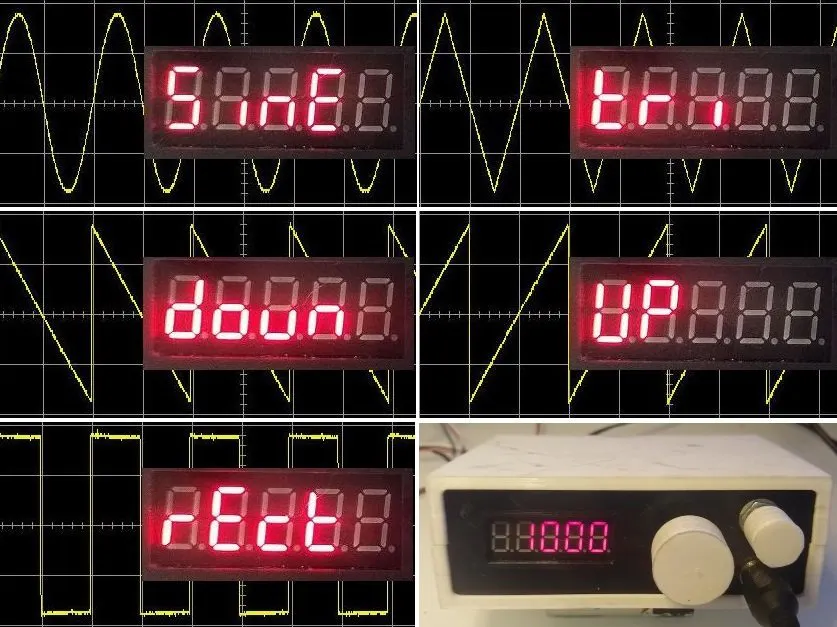## Things used in this project

### Hardware components

 Microchip ATtiny1614 See Eagle files for bill of materials
×1

### Software apps and online servicesArduino IDE

### Hand tools and fabrication machines3D Printer (generic)Soldering iron (generic)

## Custom parts and enclosures

### STL Files

STL files for 3D printing (0.2mm layer height, no supports)

## Schematics

### Schematic - Oscillator board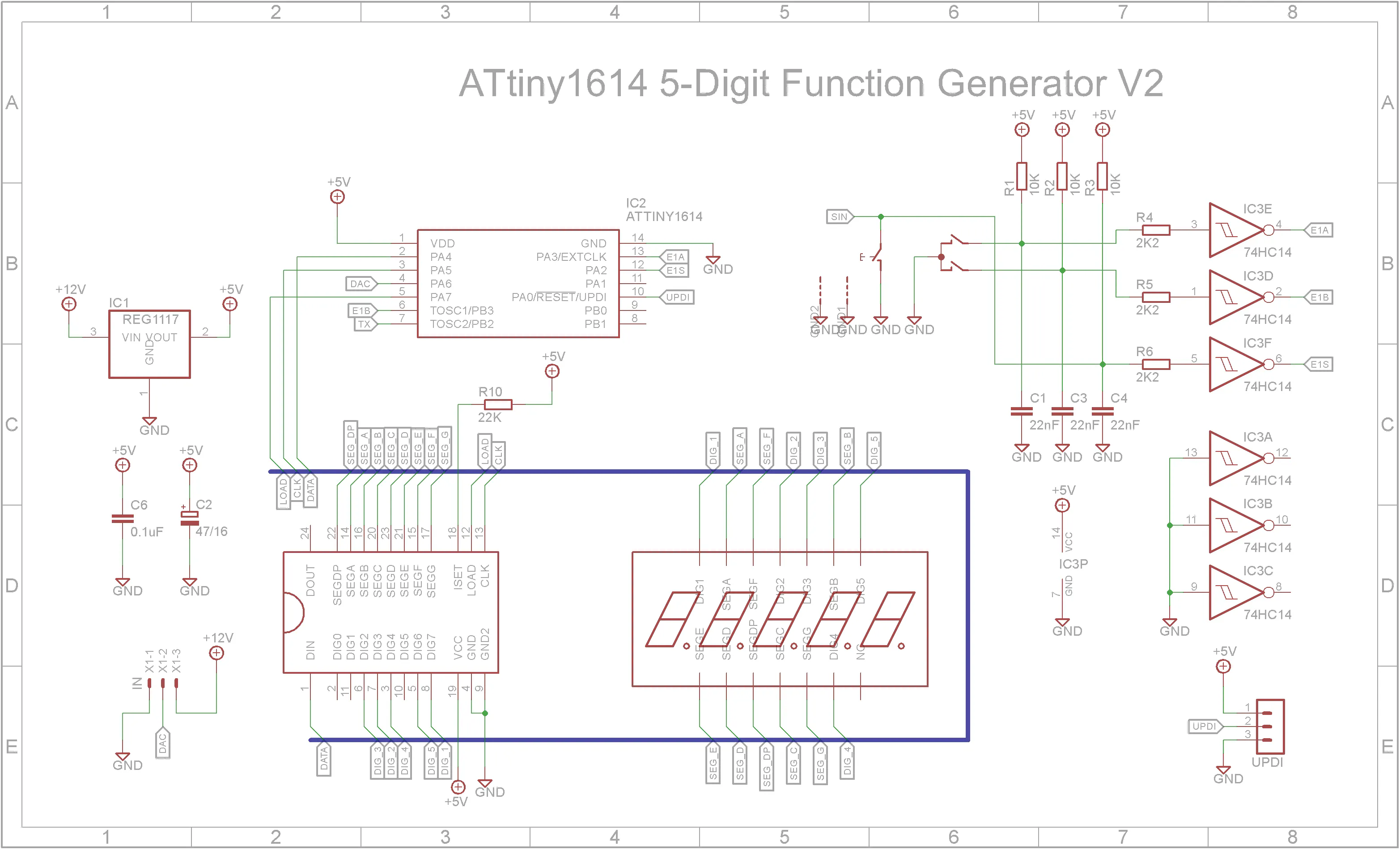### PCB - Oscillator Board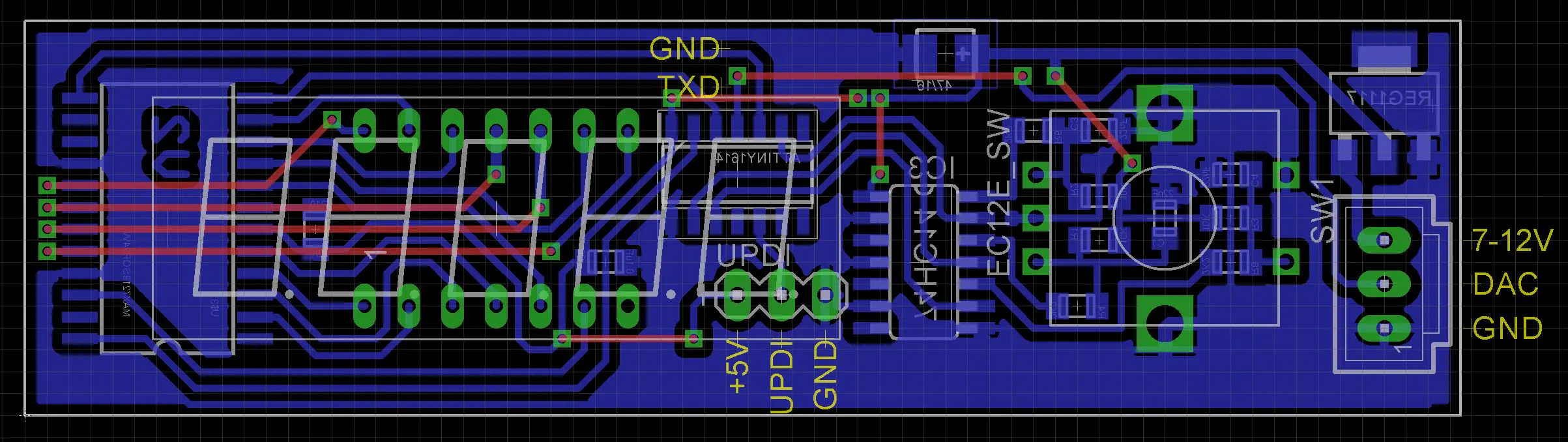### Schematic - Output Board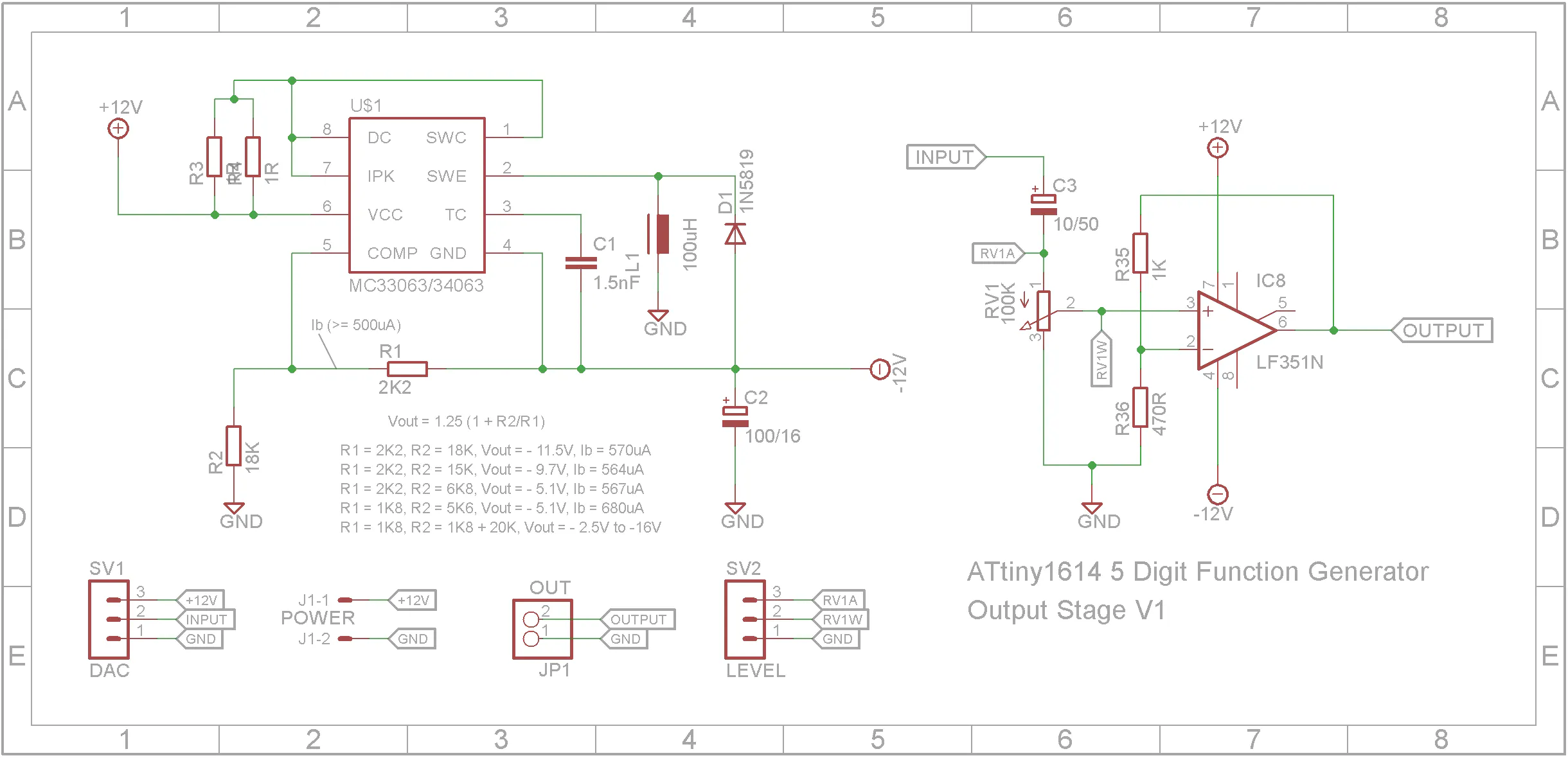### PCB - Output Board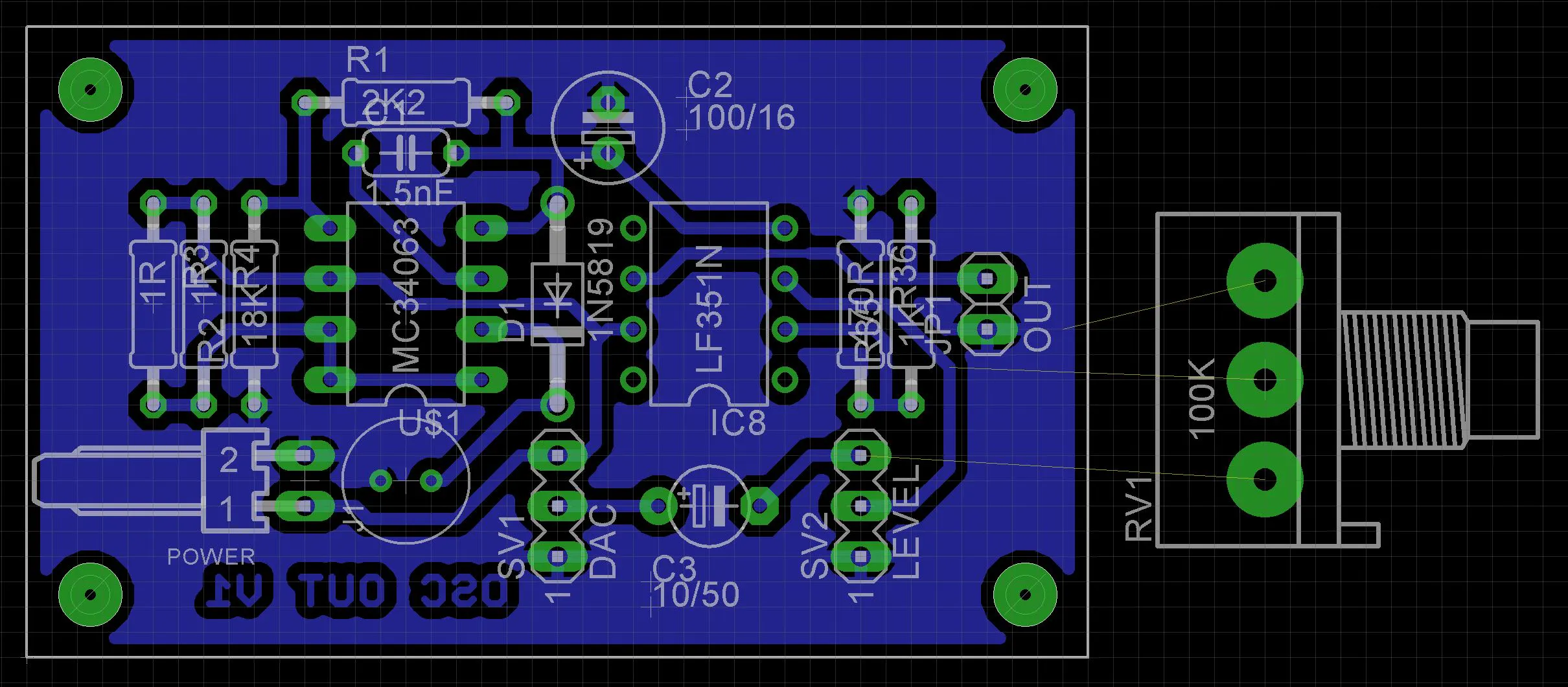### Eagle Files

Schematic and PCBs in Eagle format

## Code

### AudioOscillatorV3.ino

C/C++
```/**
* ATtiny1614 Audio Function Generator (20Hz to 20kHz)
*
* 2021-03-13 - Initial Code Base
*            - Oscillator code based on code from rgco (https://www.instructables.com/Arduino-Waveform-Generator-1/)
*            - Uses internal DAC to output waveform
* 2021-03-18 - Fix equation in calculatePhaseIncrement
* ---------------------------------------
* ATtiny1614 Pins mapped to Ardunio Pins
*
*             +--------+
*         VCC + 1   14 + GND
* (SS)  0 PA4 + 2   13 + PA3 10 (SCK)
*       1 PA5 + 3   12 + PA2 9  (MISO)
* (DAC) 2 PA6 + 4   11 + PA1 8  (MOSI)
*       3 PA7 + 5   10 + PA0 11 (UPDI)
* (RXD) 4 PB3 + 6    9 + PB0 7  (SCL)
* (TXD) 5 PB2 + 7    8 + PB1 6  (SDA)
*             +--------+
' ----------------------------------------
*/

#include <LedControl.h>

#define MAX7219_DATA 0 //PA4
#define MAX7219_CLK 1  //PA5

#define DAC_OUT 2      //PA6
#define ENC_A 10       //PA3
#define ENC_B 4        //PB3
#define ENC_S 9        //PA2

//MAX7219 not wired to correct digits on display to simply PCB routing
//Logical to Physical LED order 7,3,2,4,6
int8_t digits[] = {6, 4, 2, 3, 7};

int8_t rotaryDirection = 0;
bool lastRotA = false;
bool rotarySwitchPressed = false;

enum MenuEnum { WAVE, K10, K1, U100, U10, U1, RUN };

enum WaveEnum { SINE, TRIANGLE, RAMP_DOWN, RAMP_UP, RECTANGLE };
WaveEnum waveSelected = SINE;

#define PI 3.14159265
#define LOOP_TIME 59      //Found by trail and error. Set this to produce a 1kHz output when set to 1kHz
#define SAMPLES 256
#define DACMAX 256
byte waveform[SAMPLES];

#define FLASH_TIME 200
long flashTimeout;
int activeDigit = 0;
bool flash = false;

long currentFrequency = 1000;

//---------------------------------------------------------------------
// Setup Hardware
void setup()
{
//Encoder pins
pinMode(ENC_A,INPUT);
pinMode(ENC_B,INPUT);
pinMode(ENC_S,INPUT);

//The MAX72XX is in power-saving mode on startup, we have to do a wakeup call
lc.shutdown(0,false);
//Set the brightness to a medium values
lc.setIntensity(0,1);
//and clear the display
lc.clearDisplay(0);

//Setup the DAC
VREF.CTRLA = VREF_DAC0REFSEL_2V5_gc;
DAC0.DATA = 0;
DAC0.CTRLA = DAC_RUNSTDBY_bm | DAC_OUTEN_bm | DAC_ENABLE_bm;

//Interrupt handers for rotary encoder
attachInterrupt(ENC_A, rotaryInterrupt, CHANGE);
attachInterrupt(ENC_S, switchInterrupt, CHANGE);

}

//---------------------------------------------------------------------
// Interrupt Handler: Rotary encoder has moved
void rotaryInterrupt()
{
{
//Record direction
rotaryDirection = (digitalRead(ENC_B)) ? -1 : 1;
}
}

//---------------------------------------------------------------------
// Interrupt Handler: Rotary encoder shaft was pressed
void switchInterrupt()
{
//Record when pressed
}

//---------------------------------------------------------------------
//Display rotary control
void loop()
{
//Flash active digit
if (millis() >= flashTimeout && currentMenu != RUN)
{
flashTimeout = millis() + FLASH_TIME;
flash = !flash;

{
writeWaveform(waveSelected, flash);
}
else
{
writeNumber(currentFrequency, true, activeDigit, flash);
}
}

//Handle pressing of encoder
if (rotarySwitchPressed)
{
rotarySwitchPressed = false;

{
//Set the waveform
flashTimeout = millis();  //force update on next loop
flash = false;            //next loop will set this true
}
{
writeNumber(currentFrequency, false, 0, true);    //Show frequency
if (currentFrequency != 0)
{
setWave(waveSelected);    //Fill waveform table
runOscillator(currentFrequency);
}
}
else
{
activeDigit = 6 - (int)currentMenu;  //K10 -> 5, K1 -> 4, ... U1 = 1;
flashTimeout = millis();  //force update on next loop
flash = false;            //next loop will set this true
}
}

//Handle rotary encoder itself
if (rotaryDirection != 0)
{
{
case WAVE:
if (rotaryDirection == 1)
{
waveSelected = (waveSelected == RECTANGLE) ? SINE : (WaveEnum)((int)waveSelected + 1);
}
else
{
waveSelected = (waveSelected == SINE) ? RECTANGLE : (WaveEnum)((int)waveSelected - 1);
}
break;

case K10:
if (rotaryDirection == 1 && currentFrequency < 90000)
{
currentFrequency += 10000;
}
else if (rotaryDirection == -1 && currentFrequency > 0)
{
currentFrequency -= 10000;
}
break;

case K1:
if (rotaryDirection == 1 && currentFrequency < 99000)
{
currentFrequency += 1000;
}
else if (rotaryDirection == -1 && currentFrequency > 0)
{
currentFrequency -= 1000;
}
break;

case U100:
if (rotaryDirection == 1 && currentFrequency < 99900)
{
currentFrequency += 100;
}
else if (rotaryDirection == -1 && currentFrequency > 0)
{
currentFrequency -= 100;
}
break;

case U10:
if (rotaryDirection == 1 && currentFrequency < 99990)
{
currentFrequency += 10;
}
else if (rotaryDirection == -1 && currentFrequency > 0)
{
currentFrequency -= 10;
}
break;

case U1:
if (rotaryDirection == 1 && currentFrequency < 99999)
{
currentFrequency += 1;
}
else if (rotaryDirection == -1 && currentFrequency > 0)
{
currentFrequency -= 1;
}
break;
}
rotaryDirection = 0;
}
delay(10);
}

//---------------------------------------------------------------------
// Calculate the phase increment.
//  2^32/16E6=268.435456
//  2^32/20E0=214.7483648
unsigned long calculatePhaseIncrement(long freq)
{
return 0.2147483648 * LOOP_TIME * freq * 1000;
}

//---------------------------------------------------------------------
//Fill waveform table with amplitude values for selected waveform
//  wave - waveforem selected
void setWave(WaveEnum wave)
{
for (int s = 0; s < SAMPLES; ++s)
{
float phip = (s + 0.5) / SAMPLES;
float phi = 2 * PI * phip;
int val=0;

//sine
switch(wave)
{
case SINE: val = (sin(phi) + 1.0) * DACMAX / 2; break;
case TRIANGLE: val = abs(DACMAX * (1.0 - 2.0 * phip)); break;
case RAMP_DOWN: val = DACMAX * (1.0 - phip); break;
case RAMP_UP: val = DACMAX * phip; break;
case RECTANGLE: val = (DACMAX - 1) * (phip > 0.5); break;
}
waveform[s]=(byte)min(max(val,0),DACMAX - 1);
}
}

//---------------------------------------------------------------------
// Run oscillator
//  Run the oscillator until the rotary button pressed
__attribute__((optimize("O0")))
void runOscillator(long freq)
{
int redPhase;
unsigned long phase = 0;
unsigned long phaseInc = calculatePhaseIncrement(freq);

TCD0.INTCTRL = 0;  //Disable TCD interrupts (millis() function timer)
while (!rotarySwitchPressed)
{
phase += phaseInc;
redPhase = phase >> 24;
DAC0.DATA = waveform[redPhase];
}
TCD0.INTCTRL = TCD_OVF_bm;  //Enable TCD interrupts
}

//---------------------------------------------------------------------
//Display the current waveform on display
// s - Shape (0 - 4)
// on - true to show waveform, false to hide
void writeWaveform(WaveEnum s, bool on)
{
//Clear display
for (int i = 0; i < 5; i++)
{
lc.setChar(0, digits[i], ' ', false);
}
if (on)
{
//bit mapping to segments are dp,a,b,c, d,e,f,g
switch (s)
{
case SINE:
lc.setDigit(0, digits, 5, false);
lc.setRow(0, digits, 0x10);    //i
lc.setRow(0, digits, 0x15);    //n
lc.setChar(0, digits, 'E', false);
break;

case TRIANGLE:
lc.setRow(0, digits, 0x0F);    //t
lc.setRow(0, digits, 0x05);    //r
lc.setRow(0, digits, 0x10);    //i
break;

case RAMP_DOWN:
lc.setChar(0, digits, 'd', false);
lc.setRow(0, digits, 0x1d);    //o
lc.setRow(0, digits, 0x1c);    //u
lc.setRow(0, digits, 0x15);    //n
break;

case RAMP_UP:
lc.setRow(0, digits, 0x3E);    //U
lc.setRow(0, digits, 0x67);    //P
break;

case RECTANGLE:
lc.setRow(0, digits, 0x05);    //r
lc.setChar(0, digits, 'E', false);
lc.setRow(0, digits, 0x0d);    //c
lc.setRow(0, digits, 0x0F);    //t
break;
}
}
}

//---------------------------------------------------------------------
//Write number to display
//  num - (0 to 99999)
//  flashDigit - Digit to flash (0 - no flash, 1..6 digit position)
//  flash - true to show digit, false to show blank
void writeNumber(long num, bool leadingZeros, int flashDigit, bool flash)
{
num = max(min(num, 99999), 0);
for (int i = 0; i < 5; i++)
{
if (flashDigit == (i + 1) && !flash)
{
lc.setChar(0, digits[i], ' ', false);
}
else if (num > 0 || i == 0 || leadingZeros)
{
lc.setDigit(0, digits[i], num % 10, false);
}
else
{
lc.setChar(0, digits[i], ' ', false);
}
num = num / 10;
}
}
```